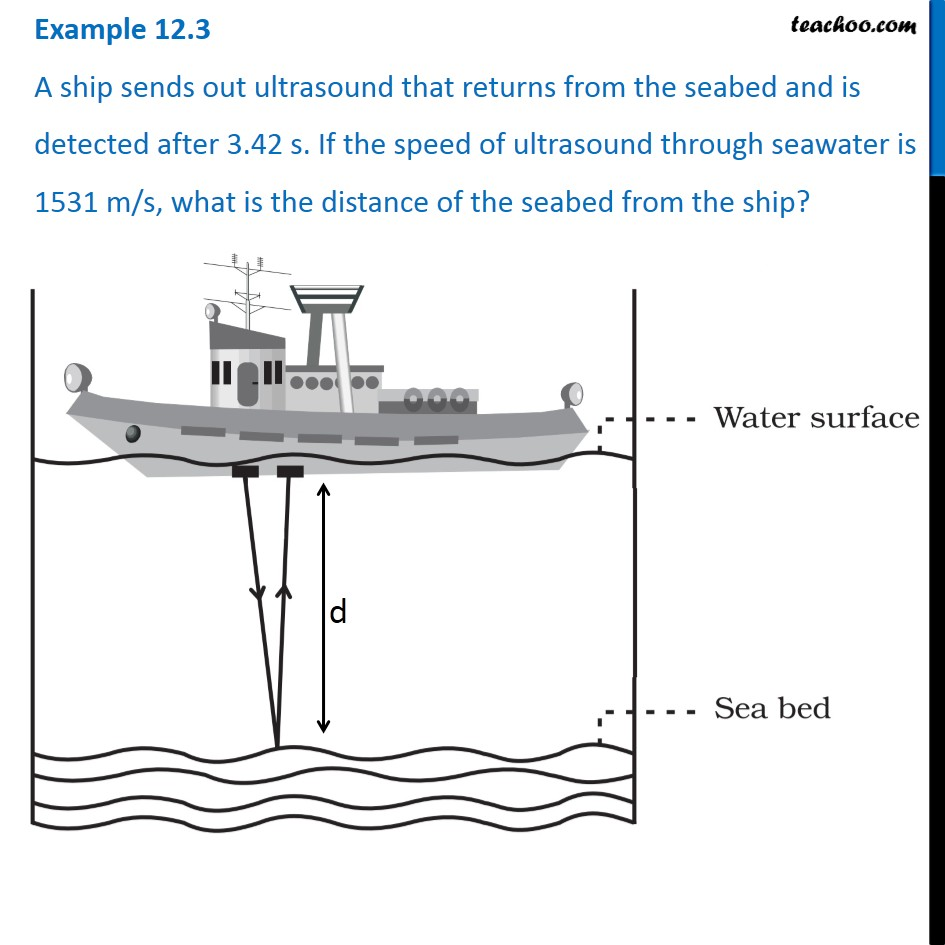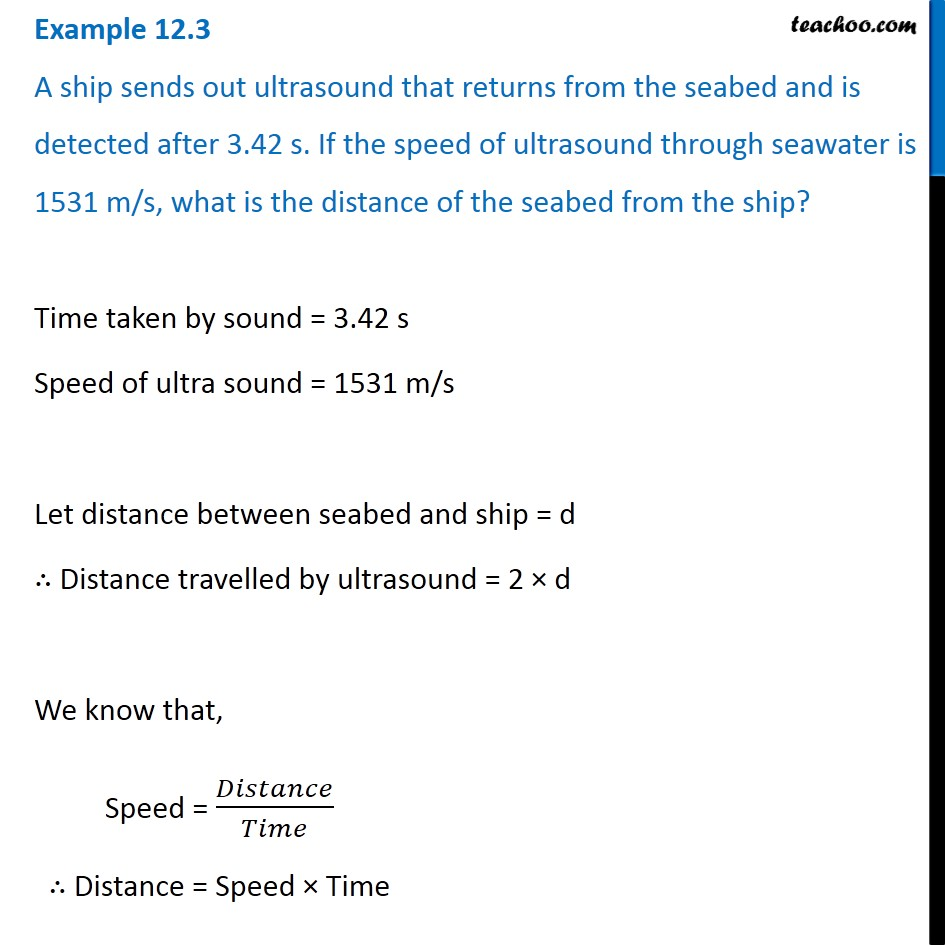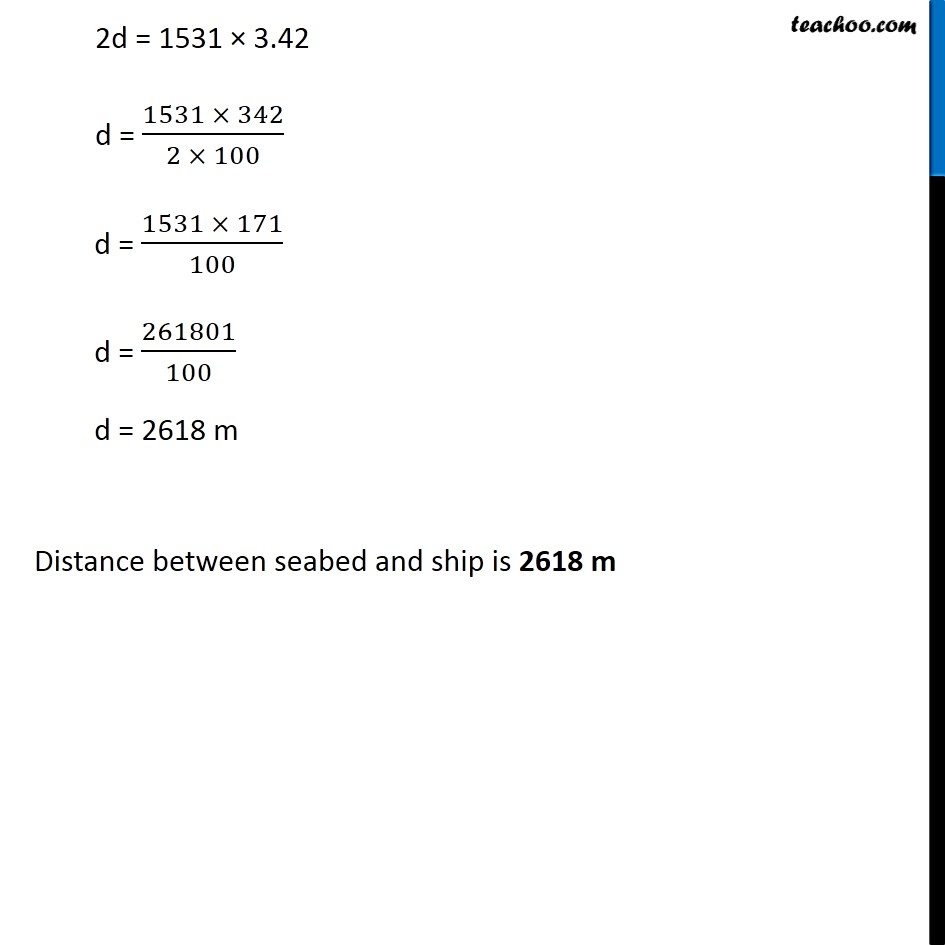Examples from NCERT Book

Class 9
Chapter 12 Class 9 - SoundLearn in your speed, with individual attention - Teachoo Maths 1-on-1 Class

### Transcript

Example 12.3 A ship sends out ultrasound that returns from the seabed and is detected after 3.42 s. If the speed of ultrasound through seawater is 1531 m/s, what is the distance of the seabed from the ship? Time taken by sound = 3.42 s Speed of ultra sound = 1531 m/s Let distance between seabed and ship = d ∴ Distance travelled by ultrasound = 2 × d We know that, Speed = 𝐷𝑖𝑠𝑡𝑎𝑛𝑐𝑒/𝑇𝑖𝑚𝑒 ∴ Distance = Speed × Time 2d = 1531 × 3.42 d = (1531 × 342)/(2 × 100) d = (1531 × 171)/100 d = 261801/100 d = 2618 m Distance between seabed and ship is 2618 m